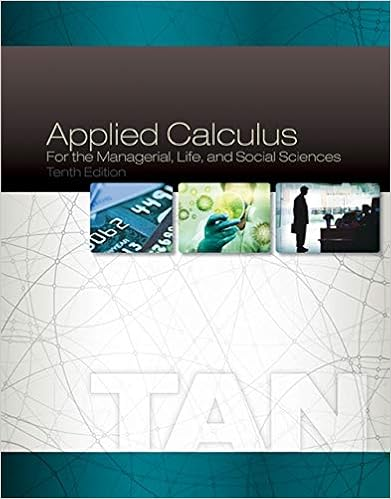# A sample of oxygen gas has its absolute temperature

• Notes
• 18
• 100% (10) 10 out of 10 people found this document helpful

This preview shows page 5 - 8 out of 18 pages.

##### We have textbook solutions for you!
The document you are viewing contains questions related to this textbook.The document you are viewing contains questions related to this textbook.
Chapter 12 / Exercise 3
Applied Calculus for the Managerial, Life, and Social Sciences
TanExpert Verified
23.A sample of oxygen gas has its absolute temperature halved while the pressure of the gas remained Econstant. If the initial volume is 400 mL, what is the final volume?a.20 mLb.133 mLc.200 mLd.400 mLe.800 mL
24.A sample container of carbon monoxide occupies a volume of 435 mL at a pressure of 785 torr and a Mtemperature of 298 K. What would its temperature be if the volume were changed to 265 mL at a pressure of 785 torr?
25.A 0.850-mole sample of nitrous oxide, a gas used as an anesthetic by dentists, has a volume of 20.46 L at M123C and 1.35 atm. What would be its volume at 468C and 1.35 atm?
26.A sample of ammonia gas at 65.5C and 524 torr has a volume of 15.31 L. What is its volume when the Mtemperature is –15.8C and its pressure is 524 torr?
69
##### We have textbook solutions for you!
The document you are viewing contains questions related to this textbook.The document you are viewing contains questions related to this textbook.
Chapter 12 / Exercise 3
Applied Calculus for the Managerial, Life, and Social Sciences
TanExpert Verified
27.A 500-mL sample of argon at 800 torr has its absolute temperature quadrupled. If the volume remains Eunchanged what is the new pressure?a.200 torrb.400 torrc.800 torrd.2400 torre.3200 torr
28.A 750-mL sample of hydrogen exerts a pressure of 822 torr at 325 K. What pressure does it exert if the Etemperature is raised to 475 K at constant volume?
29.A sample of methane gas, CH4(g), occupies a volume of 60.3 L at a pressure of 469 torr and a Mtemperature of 29.3C. What would be its temperature at a pressure of 243 torr and volume of 60.3 L?
C
30.What are the conditions of STP?E
31.A sample of propane, a component of LP gas, has a volume of 35.3 L at 315 K and 922 torr. What is its Mvolume at STP?a.25.2 Lb.30.6 Lc.33.6 Ld.37.1 Le.49.2 L
32.Nitrogen dioxide is a red-brown gas that is responsible for the color of photochemical smog. A sample of Mnitrogen dioxide has a volume of 28.6 L at 45.3C and 89.9 kPa. What is its volume at STP?
33.Calculate the pressure of a helium sample at –207.3C and 768 mL if it exerts a pressure of 175 kPa at M25.0C and 925 mL.
34.Calculate the temperature of an argon sample at 55.4 kPa and 18.6 L if it occupies 25.8 L at 75.0C and M41.1 kPa.
•••